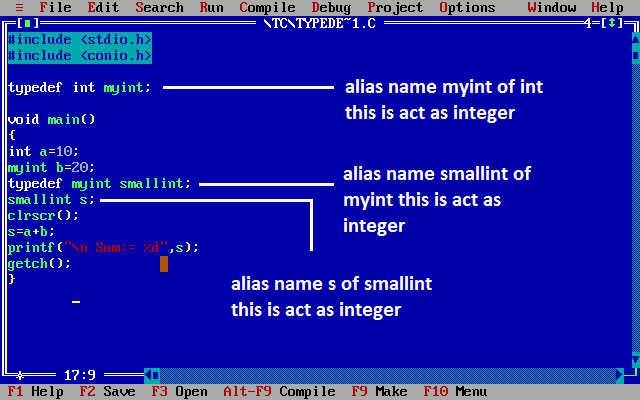# Typedef in C

## Typedef in C

The C programming language provides a keyword called typedef, by using this keyword you can create a user defined name for existing data type. Generally typedef are use to create an alias name (nickname).

## Syntax of Typedef in C

```
typedef  datatype alias_name;
```

## Example of Typedef in C

```
typedef  int Intdata;
```

## Example of typedef

```#include<stdio.h>
#include<conio.h>

typedef int Intdata; // Intdata is alias name of int
void main()
{
int a=10;
Integerdata b=20;
typedef Intdata Integerdata; // Integerdata is again alias name of Intdata
Integerdata s;
clrscr();
s=a+b;
printf("\n Sum:= %d",s);
getch();
}
```

## Output

```
Sum: 20
```

### Code Explanation

• In above program Intdata is an user defined name or alias name for an integer data type.
• All properties of the integer will be applied on Intdata also.
• Integerdata is an alias name to existing user defined name called Intdata.

Note: By using typedef only we can create the alias name and it is under control of compiler.

You can in another example, here myint is alias name for integer data and again smallint is alias name for myint.